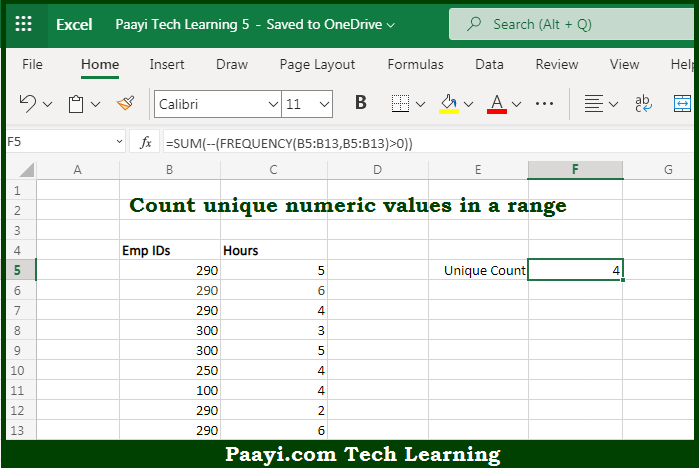# Learn How to COUNT Unique Numeric Values in Range in Microsoft Excel

Written by | 0 Comments | 557 Views

In this article, you will learn how to COUNT various things in Microsoft Excel using a single or combination of functions and its purpose. You will get to know how to COUNT Unique Numeric Values in Range and see the generic formula.

COUNT Unique Numeric Values in Range in Microsoft Excel

The main purpose of this formula is to count unique numeric values in a range. Here we will learn how to count unique numeric values in the given range in Microsoft Excel. That implies, with the help of a formula based on the FREQUENCY and SUM function you can able to count unique numeric values in a range. So, with the help of this formula, you can able to count unique numeric values in the given range.

General Formula to COUNT Unique Numeric Values in Range

=SUM(--(FREQUENCY(data,data)>0))

The Explanation for the COUNT Unique Numeric Values in RangeSo we know that with the help of the given formula above you can able to count unique numeric values in a range. Here we will learn how to count unique numeric values in the given range in Microsoft Excel. As we know that usually, The Microsoft Excel FREQUENCY function returns a frequency distribution. The result is a summary table showing the frequency of numeric values, organized in "bins". We are using here it as a roundabout method to count unique numeric values. So, with the help of this formula, you can able to count unique numeric values in a range.